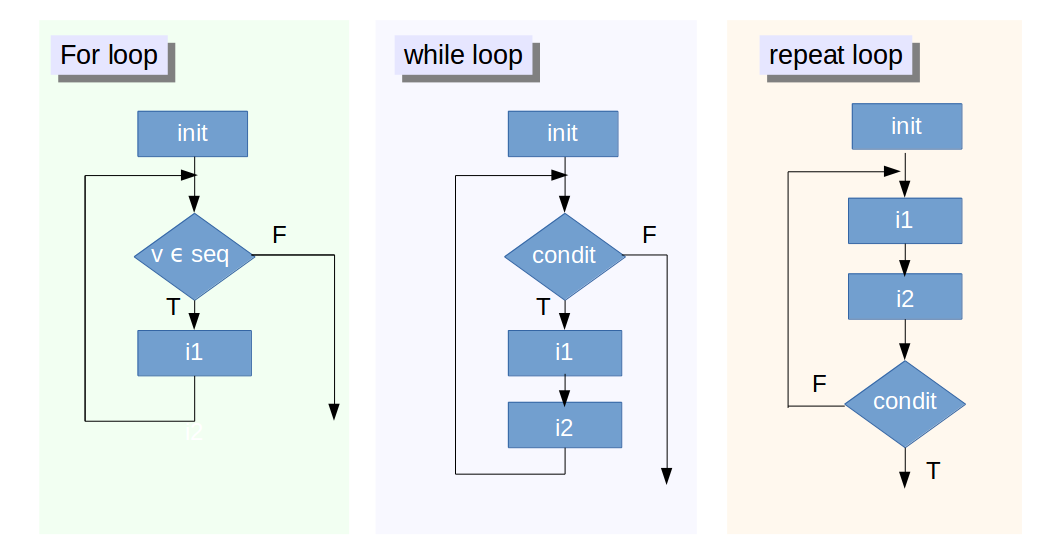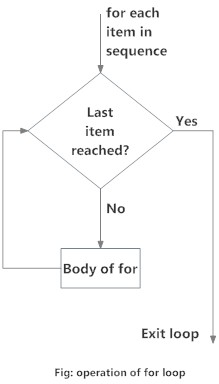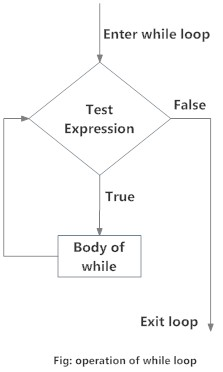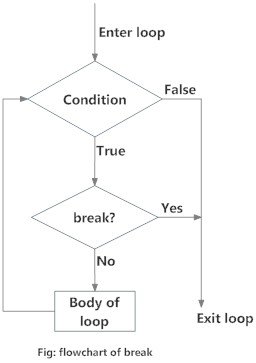# Looping in R

## What is a loop?

Process of iteration:

• automating a certain multi step process by organizing sequences of actions (‘batch’ processes)
• grouping the parts in need of repetition

### When do you know you need a loop?

#### -> When you seem to be repeating the same code.

Let’s have a look at some data and code:

air.data <- airquality
head(air.data)
##   Ozone Solar.R Wind Temp Month Day
## 1    41     190  7.4   67     5   1
## 2    36     118  8.0   72     5   2
## 3    12     149 12.6   74     5   3
## 4    18     313 11.5   62     5   4
## 5    NA      NA 14.3   56     5   5
## 6    28      NA 14.9   66     5   6

Say I wanted to calculate the mean of each column in the air.data data.frame. When I first started coding I would have probably done something like this:

mean.1 <- mean(air.data[,1], na.rm = T)
mean.2 <- mean(air.data[,2], na.rm = T)
mean.3 <- mean(air.data[,3], na.rm = T)
mean.4 <- mean(air.data[,4], na.rm = T)
mean.5 <- mean(air.data[,5], na.rm = T)
mean.6 <- mean(air.data[,1], na.rm = T)

means <- c(mean.1, mean.2, mean.3, mean.4, mean.4, mean.6)

means
##   42.129310 185.931507   9.957516  77.882353  77.882353  42.129310

## Types of loops

• for loops: Loops that execute for a prescribed number of times.
• controlled by a counter or an index
• incremented at each iteration cycle
• while or repeat loops: Loops based on the onset and verification of a logical condition (for example, the value of a control variable)
• tested at the start (while) or at the end (repeat) of the loop construct.## for loops

for loops are used when the number of iterations required can be defined: eg iterating a calculation across each row of a data.frame.General construct of a for loop:

for (val in sequence) {
statement
}

Say we wanted to scale and center each variable in the air.data dataset. We can iterate the process over of each column of the dataframe in a number of ways.

#### 1. We can use numeric indices:

for(i in 1:ncol(air.data)){
air.data[,i] <- scale(air.data[,i], scale = T, center = T)
}

head(air.data)    
##         Ozone     Solar.R       Wind       Temp     Month       Day
## 1 -0.03423409  0.04517615 -0.7259482 -1.1497140 -1.407294 -1.670019
## 2 -0.18580489 -0.75430487 -0.5556388 -0.6214670 -1.407294 -1.557210
## 3 -0.91334473 -0.41008388  0.7500660 -0.4101682 -1.407294 -1.444401
## 4 -0.73145977  1.41095624  0.4378323 -1.6779609 -1.407294 -1.331592
## 5          NA          NA  1.2326091 -2.3118573 -1.407294 -1.218782
## 6 -0.42831817          NA  1.4029185 -1.2553634 -1.407294 -1.105973

#### 2. We can use character indices:

for(var in names(air.data)){
air.data[,var] <- scale(air.data[,var], scale = T, center = T)
}

head(air.data)
##         Ozone     Solar.R       Wind       Temp     Month       Day
## 1 -0.03423409  0.04517615 -0.7259482 -1.1497140 -1.407294 -1.670019
## 2 -0.18580489 -0.75430487 -0.5556388 -0.6214670 -1.407294 -1.557210
## 3 -0.91334473 -0.41008388  0.7500660 -0.4101682 -1.407294 -1.444401
## 4 -0.73145977  1.41095624  0.4378323 -1.6779609 -1.407294 -1.331592
## 5          NA          NA  1.2326091 -2.3118573 -1.407294 -1.218782
## 6 -0.42831817          NA  1.4029185 -1.2553634 -1.407294 -1.105973

## while loops

while loops can be used when the exact number of iterations is not known a priori, for example when calculating the convergence of a cost function.General construct of a while loop:

while (test_expression) {
statement
}

Example of a while loop

i <- 1

while (i < 6) {
print(i)
i = i+1
}
##  1
##  2
##  3
##  4
##  5

### nested loops

We can even nest loops within loops.

mat = matrix(nrow=5, ncol=5) # create a 30 x 30 matrix (of 30 rows and 30 columns)

for(i in 1:nrow(mat))  # for each row
{
for(j in 1:ncol(mat)) # for each column
{
mat[i,j] = i*j     # assign values based on position: product of two indexes
}
}

i iterates over each row while j interates over each column. What have we made? The all too familiar multiplication table!

mat
##      [,1] [,2] [,3] [,4] [,5]
## [1,]    1    2    3    4    5
## [2,]    2    4    6    8   10
## [3,]    3    6    9   12   15
## [4,]    4    8   12   16   20
## [5,]    5   10   15   20   25

## collecting the output of loops

### individual values

Individual values can be collected in a named vector by combining functions c() and setNames().

For example we could collect the means of each column of the air.data data.frame in a vector.

mu <- NULL

for(var in names(air.data)){
mu <- c(mu, setNames(mean(air.data[,var], na.rm = T), var))
}

mu
##      Ozone    Solar.R       Wind       Temp      Month        Day
##  42.129310 185.931507   9.957516  77.882353   6.993464  15.803922

### vectors

Vectors of the same size can be collected in a data.frame.

In the scaling example before we were iterating through the air.data data.frame and overwriting the original values. But what if we wanted to retain the original data. We then need to collect the outputs in a new data.frame.

require(dplyr)

scaled_data <- NULL

for(var in names(air.data)){

scaled_data <- cbind(scaled_data,
scale(air.data[,var],
scale = T,
center = T))
}

# convert to data.frame and name
scaled_data <- as.data.frame(scaled_data) %>% setNames(names(air.data))

head(scaled_data)
##         Ozone     Solar.R       Wind       Temp     Month       Day
## 1 -0.03423409  0.04517615 -0.7259482 -1.1497140 -1.407294 -1.670019
## 2 -0.18580489 -0.75430487 -0.5556388 -0.6214670 -1.407294 -1.557210
## 3 -0.91334473 -0.41008388  0.7500660 -0.4101682 -1.407294 -1.444401
## 4 -0.73145977  1.41095624  0.4378323 -1.6779609 -1.407294 -1.331592
## 5          NA          NA  1.2326091 -2.3118573 -1.407294 -1.218782
## 6 -0.42831817          NA  1.4029185 -1.2553634 -1.407294 -1.105973

### other

more complex outputs can be collected in lists. For example, say we wanted to fit a linear model with "Ozone" as the response variable and each of the other variables as single predictors. We can loop the process and collect the outputs of the lm() function in a list.

predictors <- names(air.data)[names(air.data) != "Ozone"]
air_mods <- NULL

for(predictor in predictors){

air_mods <- c(air_mods,
list(lm(as.formula(paste("Ozone ~", predictor)), data = air.data)))
}

str(air_mods, max.level = 1)
## List of 5
##  $:List of 13 ## ..- attr(*, "class")= chr "lm" ##$ :List of 13
##   ..- attr(*, "class")= chr "lm"
##  $:List of 13 ## ..- attr(*, "class")= chr "lm" ##$ :List of 13
##   ..- attr(*, "class")= chr "lm"
##  \$ :List of 13
##   ..- attr(*, "class")= chr "lm"

## Altering looping sequences

### break

break is a bit like stop() but for a loop. It is usually used with a conditional statement and if triggered, breaks out of the current loop.x <- 1:5

for (val in x) {
if (val == 3){
break
}
print(val)
}
##  1
##  2

In this example, we iterate over the vector x, which has consecutive numbers from 1 to 5. Inside the for loop we have used a condition to break if the current value is equal to 3. As we can see from the output, the loop terminates when it encounters the break statement.

## vectorisation (the apply function family)

It’s always good to start learning the principles of iteration through loops. Simple loops can be more understandable to a human reader

However, loops can be slow, and in cases were computation time of a loop becomes a bottleneck, it is good to know a bit about vectorisation.

So let’s see what our for loop examples look like vectorised:

### apply

apply iterates over the margins of an array. We can use it to calculate the means of each column:

mu <- apply(air.data, MARGIN = 2, FUN = function(x){mean(x, na.rm = T)})

### lapply

The lapply takes a list as an input (data.frames are lists) and apply a function over each element of the list:

scaled_data <- lapply(air.data, FUN = function(x){
scale(x, scale = T, center = T)}) %>%
data.frame() %>%
setNames(names(air.data))

head(scaled_data)
##         Ozone     Solar.R       Wind       Temp     Month       Day
## 1 -0.03423409  0.04517615 -0.7259482 -1.1497140 -1.407294 -1.670019
## 2 -0.18580489 -0.75430487 -0.5556388 -0.6214670 -1.407294 -1.557210
## 3 -0.91334473 -0.41008388  0.7500660 -0.4101682 -1.407294 -1.444401
## 4 -0.73145977  1.41095624  0.4378323 -1.6779609 -1.407294 -1.331592
## 5          NA          NA  1.2326091 -2.3118573 -1.407294 -1.218782
## 6 -0.42831817          NA  1.4029185 -1.2553634 -1.407294 -1.105973

### mapply

The mapply allows us to pass multiple iterated arguments to a function. It has a different structure as the function is the first argument, any arguments to be passed to the function and iterated over are specified in ... and any arguments to be used as is by the function are supplied in the argument MoreArgs.

As an example, we will replicate the default behaviour of scale to center on the mean by supply are own vector of calculated means mu.

scaled_data <- mapply(FUN = function(x, center){scale(x, scale = T,
center = center)},
x = air.data, center = mu) %>%
data.frame() %>%
setNames(names(air.data))

head(scaled_data)
##         Ozone     Solar.R       Wind       Temp     Month       Day
## 1 -0.03423409  0.04517615 -0.7259482 -1.1497140 -1.407294 -1.670019
## 2 -0.18580489 -0.75430487 -0.5556388 -0.6214670 -1.407294 -1.557210
## 3 -0.91334473 -0.41008388  0.7500660 -0.4101682 -1.407294 -1.444401
## 4 -0.73145977  1.41095624  0.4378323 -1.6779609 -1.407294 -1.331592
## 5          NA          NA  1.2326091 -2.3118573 -1.407294 -1.218782
## 6 -0.42831817          NA  1.4029185 -1.2553634 -1.407294 -1.105973

### Things to remember:

• loops are evaluated in the global environment -> can get messy!
• if you’ve got a lot of code within your loop, consider writing a function

### Cheats

A lot of the examples I showed are actually addressed by these or other functions.

eg scale() can be applied directly to a data.frame:

head(scale(air.data))
##            Ozone     Solar.R       Wind       Temp     Month       Day
## [1,] -0.03423409  0.04517615 -0.7259482 -1.1497140 -1.407294 -1.670019
## [2,] -0.18580489 -0.75430487 -0.5556388 -0.6214670 -1.407294 -1.557210
## [3,] -0.91334473 -0.41008388  0.7500660 -0.4101682 -1.407294 -1.444401
## [4,] -0.73145977  1.41095624  0.4378323 -1.6779609 -1.407294 -1.331592
## [5,]          NA          NA  1.2326091 -2.3118573 -1.407294 -1.218782
## [6,] -0.42831817          NA  1.4029185 -1.2553634 -1.407294 -1.105973

and there are in-built functions for calculating the mean of columns:

colMeans(air.data, na.rm = T)
##      Ozone    Solar.R       Wind       Temp      Month        Day
##  42.129310 185.931507   9.957516  77.882353   6.993464  15.803922

But the principles of applying functions over loops are still the same.

### Exercises

#### source: http://r-exercises.com/2016/06/01/scripting-loops-in-r/

Exercise 3

With, i <- 1, write a while() loop that prints the odd numbers from 1 through 7.

Exercise 4

Using the following variables:

msg <- c(“Hello”) i <- 1

Write a while() loop that increments the variable, i, 6 times, and prints msg at every iteration.

Exercise 5

Write a for() loop that prints the first four numbers of this sequence:

x <- c(7, 4, 3, 8, 9, 25)

Exercise 6

For the next exercise, write a for() loop that prints all the letters in:

y <- c("q", "w", "e", "r", "z", "c")

Exercise 7

Using i <- 1, write a while() loop that prints the variable, i, (that is incremented from 1 – 5), and uses break to exit the loop if i equals 3.

Exercise 8

Write a nested loop, where the outer for() loop increments a 3 times, and the inner for() loop increments b 3 times. The break statement exits the inner for() loop after 2 incrementations. The nested loop prints the values of variables, a and b.

Exercise 9

Write a while() loop that prints the variable, i, that is incremented from 2 – 5, and uses the next statement, to skip the printing of the number 3.

Exercise 10

Write a for() loop that uses next to print all values except 3 in the following variable: i <- 1:5